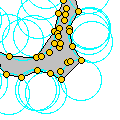CGAL 5.5.2 - 2D Alpha Shapes
2D Alpha Shapes ReferenceTran Kai Frank Da
This package offers a data structure encoding the whole family of alpha-complexes related to a given 2D Delaunay or regular triangulation. In particular, the data structure allows to retrieve the alpha-complex for any alpha value, the whole spectrum of critical alpha values and a filtration on the triangulation faces (this filtration is based on the first alpha value for which each face is included on the alpha-complex).
Introduced in: CGAL 2.1
Depends on: 2D Triangulations
BibTeX: cgal:d-as2-23a
Windows Demo: 2D Alpha Shapes
Common Demo Dlls: dlls

This chapter presents a framework for alpha shapes. The description is based on the articles , . Alpha shapes are the generalization of the convex hull of a point set. Let $$S$$ be a finite set of points in $$\mathbb{R}^d$$, $$d = 2,3$$ and $$\alpha$$ a parameter with $$0 \leq \alpha \leq \infty$$. For $$\alpha = \infty$$, the $$\alpha$$-shape is the convex hull of $$S$$. As $$\alpha$$ decreases, the $$\alpha$$-shape shrinks and develops cavities, as soon as a sphere of radius $$\sqrt{\alpha}$$ can be put inside. Finally, for $$\alpha = 0$$, the $$\alpha$$-shape is the set $$S$$ itself.

We distinguish two versions of alpha shapes, one is based on the Delaunay triangulation and the other on its generalization, the regular triangulation, replacing the natural distance by the power to weighted points. The metric used determines an underlying triangulation of the alpha shape and thus, the version computed. The basic alpha shape (cf. Example for Basic Alpha Shapes) is associated with the Delaunay triangulation (cf. Section Delaunay Triangulations). The weighted alpha shape (cf. Example for Weighted Alpha Shapes ) is associated with the regular triangulation (cf. Section Regular Triangulations).

There is a close connection between alpha shapes and the underlying triangulations. More precisely, the $$\alpha$$-complex of $$S$$ is a subcomplex of this triangulation of $$S$$, containing the $$\alpha$$-exposed $$k$$-simplices, $$0 \leq k \leq d$$. A simplex is $$\alpha$$-exposed, if there is an open disk (resp. ball) of radius $$\sqrt{\alpha}$$ through the vertices of the simplex that does not contain any other point of $$S$$, for the metric used in the computation of the underlying triangulation. The corresponding $$\alpha$$-shape is defined as the underlying interior space of the $$\alpha$$-complex.

In general, an $$\alpha$$-complex is a non-connected and non-pure polytope, meaning that a $$k$$-simplex, with $$0 \leq k \leq d-1$$, is not necessarily adjacent to a $$(k+1)$$-simplex.

The $$\alpha$$-shapes of $$S$$ form a discrete family, even though they are defined for all real numbers $$\alpha$$ with $$0 \leq \alpha \leq \infty$$. Thus, we can represent the entire family of $$\alpha$$-shapes of $$S$$ by the underlying triangulation of $$S$$. In this representation each $$k$$-simplex of the underlying triangulation is associated with an interval that specifies for which values of $$\alpha$$ the $$k$$-simplex belongs to the $$\alpha$$-shape. Relying on this result, the family of $$\alpha$$-shapes can be computed efficiently and relatively easily. Furthermore, we can select an appropriate $$\alpha$$-shape from a finite number of different $$\alpha$$-shapes and corresponding $$\alpha$$-values.

## Concepts

• AlphaShapeTraits_2
• WeightedAlphaShapeTraits_2
• AlphaShapeFace_2
• AlphaShapeVertex_2

## Classes

• CGAL::Alpha_shape_2<Dt>
• CGAL::Alpha_shape_vertex_base_2<AlphaShapeTraits_2>
• CGAL::Alpha_shape_face_base_2<AlphaShapeTraits_2, TriangulationFaceBase_2>

Concepts

## Classes

class  CGAL::Alpha_shape_2< Dt, ExactAlphaComparisonTag >
The class Alpha_shape_2 represents the family of $$\alpha$$-shapes of points in a plane for all positive $$\alpha$$. More...

class  CGAL::Alpha_shape_face_base_2< Traits, Fb, ExactAlphaComparisonTag >
The class Alpha_shape_face_base_2 is the default model for the concept AlphaShapeFace_2. More...

class  CGAL::Alpha_shape_vertex_base_2< Traits, Vb, ExactAlphaComparisonTag >
The class Alpha_shape_vertex_base_2 is the default model for the concept AlphaShapeVertex_2. More...